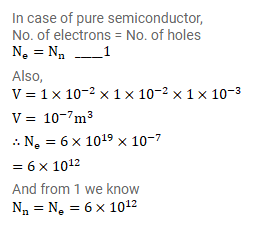# In a pure semiconductor, the number of conduction electrons is

Question:

In a pure semiconductor, the number of conduction electrons is $6 \times 10^{19} \mathrm{Per}$ cubic mertre. How many holes are there in a sample of size $1 \mathrm{~cm} \times 1 \mathrm{~cm} \times 1 \mathrm{~cm}$ ?

Solution: